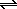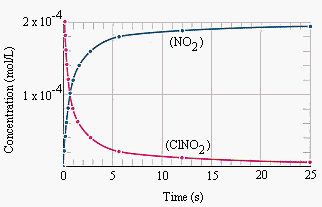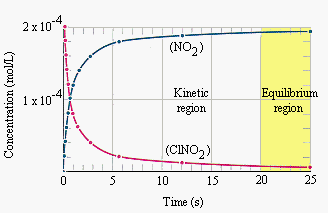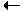Gas-Phase Reactions

Gas-Phase Reactions

The simplest chemical reactions are those that occur in the gas phase in a single step, such as the transfer of a chlorine atom from ClNO2 to NO to form NO2 and ClNO.

 ClNO2(g) + NO(g)NO2(g) + ClNO(g)

This reaction can be understood by writing the Lewis structures for all four components of the reaction. Both NO and NO2 contain an odd number of electrons. Both NO and NO2 can therefore combine with a neutral chlorine atom to form a molecule in which all of the electrons are paired. This reaction therefore involves the transfer of a chlorine atom from one molecule to another, as shown in the figure below.The figure below combines a plot of the disappearance of the ClNO2 consumed in this reaction with a plot of the appearance of NO2 formed in the reaction.One of the goals of collecting these data is to describe the rate of reaction, which is the rate at which the reactants are transformed into the products of the reaction.

The mathematical equation that describes the rate of a chemical reaction is called the rate law for the reaction. The data in the figure above are consistent with the following rate law for this reaction.

Rate = k(ClNO2)(NO)

According to this rate law, the rate at which ClNO2 and NO are converted into NO2 and ClNO is proportional to the product of the concentrations of the two reactants. Initially, the rate of reaction is fast. As the reactants are converted into products, however, the ClNO2 and NO concentrations become smaller, and the reaction slows down.

We might expect the reaction to stop when it runs out of either ClNO2 or NO. In practice, the reaction stops before this happens.

This is a very fast reactionthe concentration of ClNO2 drops by a factor of two in less than a second. And yet, no matter how long we wait, some residual ClNO2 and NO remains in the reaction flask.

The figure below divides the plot of the change in the concentrations of NO2 and ClNO into a kinetic region and an equilibrium region. By definition, the kinetic region is the period during which the concentrations of the components of the reaction are constantly changing. The equilibrium region is the period after which the reaction seems to stop, when there is no further change in the concentrations of the components of the reaction.A Collision Theory Model for Gas-Phase Reactions

The fact that the following reaction

 ClNO2(g) + NO(g)NO2(g) + ClNO(g)

seems to stop before all of the reactants are consumed can be explained with a model of chemical reactions known as the collision theory. This model assumes that ClNO2 and NO molecules must collide before a chlorine atom can be transferred from one molecule to the other.

This assumption explains why the rate of the reaction is proportional to the concentration of both ClNO2 and NO.

Rate = k(ClNO2)(NO)

The number of collisions per second between ClNO2 and NO molecules depends on their concentrations. As ClNO2 and NO are consumed in the reaction, the number of collisions per second between these molecules becomes smaller, and the reaction slows down.

Suppose that we start with a mixture of ClNO2 and NO, but no NO2 or ClNO. The only reaction that can occur at first is the transfer of a chlorine atom from ClNO2 to NO.

 ClNO2(g) + NO(g)NO2(g) + ClNO(g)

Eventually, NO2 and ClNO build up in the reaction flask, and these molecules begin to collide as well. Collisions between these molecules can result in the transfer of a chlorine atom in the opposite direction.

 ClNO2(g) + NO(g)NO2(g) + ClNO(g)

The collision theory model of chemical reactions assumes that the rate of a simple, one-step reaction is proportional to the product of the concentrations of the ions or molecules consumed in that reaction. The rate of the forward reaction is therefore proportional to the product of the concentrations of the two "reactants."

Rateforward = kf(ClNO2)(NO)

The rate of the reverse reaction, on the other hand, is proportional to the concentrations of the "products" of the reaction.

Ratereverse = kr(NO2)(ClNO)

Initially, the rate of the forward reaction is much larger than the rate of the reverse reaction, because the system contains ClNO2 and NO, but virtually no NO2 and ClNO.

 Initially: rateforward >> ratereverse

As ClNO2 and NO are consumed, the rate of the forward reaction slows down. At the same time, NO2 and ClNO accumulate, and the reverse reaction speeds up.

If the forward reaction gradually slows down and the reverse reaction speeds up, the system eventually has to reach a point at which the rates of the forward and reverse reactions are the same.

 Eventually: rateforward = ratereverse

At this point, the reaction will seem to stop. ClNO2 and NO will be consumed in the forward reaction at the rate at which they are produced in the reverse reaction. The same thing will happen to NO2 and ClNO. When the rates of the forward and reverse reactions are the same, there is no longer any change in the concentrations of the reactants or products of the reaction. In other words, the reaction is at equilibrium.

We can now see that there are two definitions of equilibrium.

1. A system in which there is no apparent change in the concentrations of the reactants and products of a reaction.

2. A system in which the rates of the forward and reverse reactions are equal.

The first definition is based on the results of experiments that tell us that some reactions seem to stop prematurelythey reach a point at which no more reactants are converted into products before the limiting reagent is consumed. The other definition is based on a theoretical model of chemical reactions that explains why reactions reach equilibrium.

We can now distinguish between reactions that go to completion and those that reach equilibrium. Reactions that aren't reversible, or that strongly favor the products, are assumed to go to completion and are represented by equations that contain a single arrow.

 2 Mg(s) + O2(g)2 MgO(s)

Reversible reactions that reach equilibrium are indicated by a pair of arrows between the two sides of the equation.

 ClNO2(g) + NO(g)NO2(g) + ClNO(g)